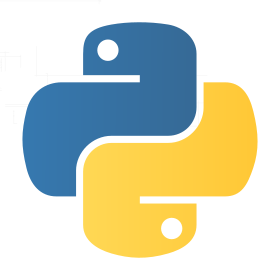# Nicholas Rinard Keene's Little Bit

I don't have a lot to say, but this is my little bit.

## Monday, September 28, 2009

### Simple Genetic Algorithm In PythonFollowing is a simple genetic algorithm written in python. A genetic algorithm uses a model of genetic evolution to find solutions to a problem which are increasingly good, until they reach a threshold of goodness. A population of beginning organisms experiences mutations and selections so that organisms with positive mutations are selected over organisms without positive mutations, or with negative mutations.

This algorithm solves a straightforward problem: finding a string. Each organism starts off with a string of correct length but having only spaces. In each generation, some of the organisms will experience mutations, which in this algorithm means one or more of the characters in their string is randomly changed. Organisms are then allowed to breed a certain number of times, then the fitness of each organism is calculated and the most fit organisms live on to the next generation. In this algorithm, the overall population count is kept steady from generation to generation, and fitness is calculated by how many characters an organism shares in common with a target string.

You can modify the parameters of this algorithm by controlling the numberOfOrganisms, chanceOfMutation, numberOfMutations, and matesPerGen. You can also change the target string to be longer, shorter, or just different; but make sure that the 'blank' string is exactly the same length, or this algorithm won't be able to finish.

I am learning python using this script, so it may not be in model python code style.

```import random

chars = ['a', 'b', 'c', 'd', 'e', 'f', 'g', 'h', 'i', 'j',
'k', 'l', 'm', 'n', 'o', 'p', 'q', 'r', 's', 't',
'u', 'v', 'w', 'x', 'y', 'z', 'A', 'B', 'C', 'D',
'E', 'F', 'G', 'H', 'I', 'J',
'K', 'L', 'M', 'N', 'O', 'P', 'Q', 'R', 'S', 'T',
'U', 'V', 'W', 'X', 'Y', 'Z',
'1', '2', '3', '4', '5', '6', '7', '8', '9', '0',
' ', ',', '.']

numberOfOrganisms = 50;
chanceOfMutation = 3 # one chance in this many
numberOfMutations = 3 # up to this many, if mutations are selected to happen at all
matesPerGen = 3; # each organism produces this many offspring for the next generation
target = """All legislative Powers herein granted shall be vested in a Congress of the United States"""
blank  = """                                                                                        """
lenTarget = len(target);

class organism:
fitness = 0
value = blank

def __cmp__( self, other ):
return cmp( other.fitness, self.fitness )

# an organism mates with another organism by comparing the chars of their values
# if the chars are equal, the offspring gets that char
# if they are not equal, the offspring gets one or the other, randomly
def mate( self, other ):
retCell = organism()
retCell.value = ""
for i in range(0,len(self.value)):
if self.value[i] == other.value[i]:
retCell.value += self.value[i]
elif random.randint(0,1) == 0:
retCell.value += self.value[i]
else:
retCell.value += other.value[i]
retCell.evaluateFitness( target )
return retCell

# this function may mutate the value of the organism in random places
def maybeMutate( self ):
if( random.randint( 0, chanceOfMutation ) == 0 ):
for i in range(random.randint(0,numberOfMutations)):
insertLoc = random.randint(0, len(self.value)-1)
self.value = self.value[0:insertLoc] + chars[random.randint(0, len(chars)-1)] + self.value[insertLoc+1:]
self.evaluateFitness( target )

# an organism's fitness is equal to the number of chars it shares with the target
def evaluateFitness( self, target ):
self.fitness = 0
for j in range(0,len(self.value)):
if self.value[j] == target[j]:
self.fitness += 1;
return self.fitness

def beginMutations( cells ):
keepGoing = True
iterations = 0
highestFitness = 0 # also an index into fitnessIterations
fitnessIterations = []

while( keepGoing ):
# a fresh next generation
iterations += 1
nextGenCells = []

# mutate the cells into a new arary
# evaluate the fitness of the mutated cells
for cell in cells:
cell.maybeMutate()
cell.evaluateFitness( target )

# mate all the cells into a new generation
# this will produce matesPerGen*len(cells) next-gen cells
for cell1 in cells:
for i in range(0, matesPerGen):
cell2 = cells[random.randint(0, len(cells)-1)]
childCell = cell1.mate( cell2 )
childCell.evaluateFitness( target )
nextGenCells.append( childCell )

# select cells into the next generation
nextGenCells.sort()

cells = nextGenCells[0:numberOfOrganisms]
if( cells.fitness > highestFitness ):
print "* new most fit *"
highestFitness = cells.fitness
for i in range( len( fitnessIterations ), highestFitness ):
fitnessIterations.append( iterations )

print "%d Most fit: %d of %d ~ %s" % (iterations, cells.fitness, lenTarget, cells.value)

#print "Selected next generation:"
for cell in cells:
#print cell.value, cell.fitness
if(cell.fitness == lenTarget):
print "!! In iteration %d found target %s" % (iterations, cell.value)
keepGoing = False

print "History of progress:"
for ind in range(len(fitnessIterations)):
print ind, fitnessIterations[ind]

# initialize the ecosystem and begin the mutations
starterCells = []
for i in range( numberOfOrganisms ):
starterCells.append( organism() )

beginMutations( starterCells )```